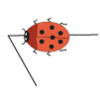# Primary Generic Proof### Two Numbers Under the Microscope

##### Age 5 to 7Challenge Level

This investigates one particular property of number by looking closely at an example of adding two odd numbers together.### Odd Times Even

##### Age 5 to 7Challenge Level

This problem looks at how one example of your choice can show something about the general structure of multiplication.### Walking Round a Triangle

##### Age 5 to 7Challenge Level

This ladybird is taking a walk round a triangle. Can you see how much he has turned when he gets back to where he started?### Take One Example

##### Age 5 to 11

This article introduces the idea of generic proof for younger children and illustrates how one example can offer a proof of a general result through unpacking its underlying structure.### Take Three Numbers

##### Age 7 to 11Challenge Level

What happens when you add three numbers together? Will your answer be odd or even? How do you know?### Square Subtraction

##### Age 7 to 11Challenge Level

Look at what happens when you take a number, square it and subtract your answer. What kind of number do you get? Can you prove it?### Round a Hexagon

##### Age 7 to 11Challenge Level

This problem shows that the external angles of an irregular hexagon add to a circle.### Three Neighbours

##### Age 7 to 11Challenge Level

Look at three 'next door neighbours' amongst the counting numbers. Add them together. What do you notice?